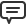# Blog

All Blog Posts  |  Next Post  |  Previous Post

#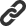Visual math formula editor for TMS Analytics

Bookmarks:

#### Tuesday, September 6, 2022

The latest versions of the TMS Analytics & Physics library introduced special components to convert analytical expressions to external formats. The new version 3.5 contains another converter component – TFNCASCII2FormulaConverter. This component is intended to convert ASCII Math formulae to analytical expressions (that can be evaluated with the Analytics core algorithms). The ASCII Math is often used as the markup language in formula editors. Thus, the TFNCASCII2FormulaConverter component brings new functionality for the library: creating complicated math formulae with external editors and then use the formulae for evaluation purposes, such as calculating values of functions, evaluating symbolic derivatives, and so on.
The converter component is very simple and has the following properties:

• ASCIIFormula (string) – a formula in ASCII Math format.
• Formula (string) – the formula in Analytics format (read-only).
• Valid (boolean) – the ASCII Math formula is valid for conversion (read-only).
• Error (string) – description of the error if occurred during conversion (read-only).
In the picture below, we showed an example of the component in design-time: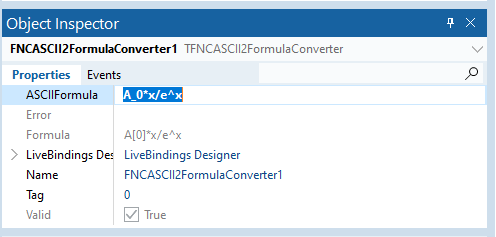The component provides the following functionality: when the ‘ASCIIFormula’ property changes, the value is converted to analytics format and assigned to the ‘Formula’ property.
Let’s create a math application that allows to input a math formula with a WYSIWYG editor, calculate its symbolic derivative and draw the graph of the functions.
In the previous articles, we described how to use FNC Chart components to draw functions with charts and evaluate symbolic derivatives of math expressions. We’ll use the same components for this application.
As a WYSIWYG math editor, we’ll use the TTMSFNCMathEditor component from the TMS FNC WX Pack. We need two editors for our application: one to input the formula of the function, another to show the expression of the derivative. The design of the form is in the picture below: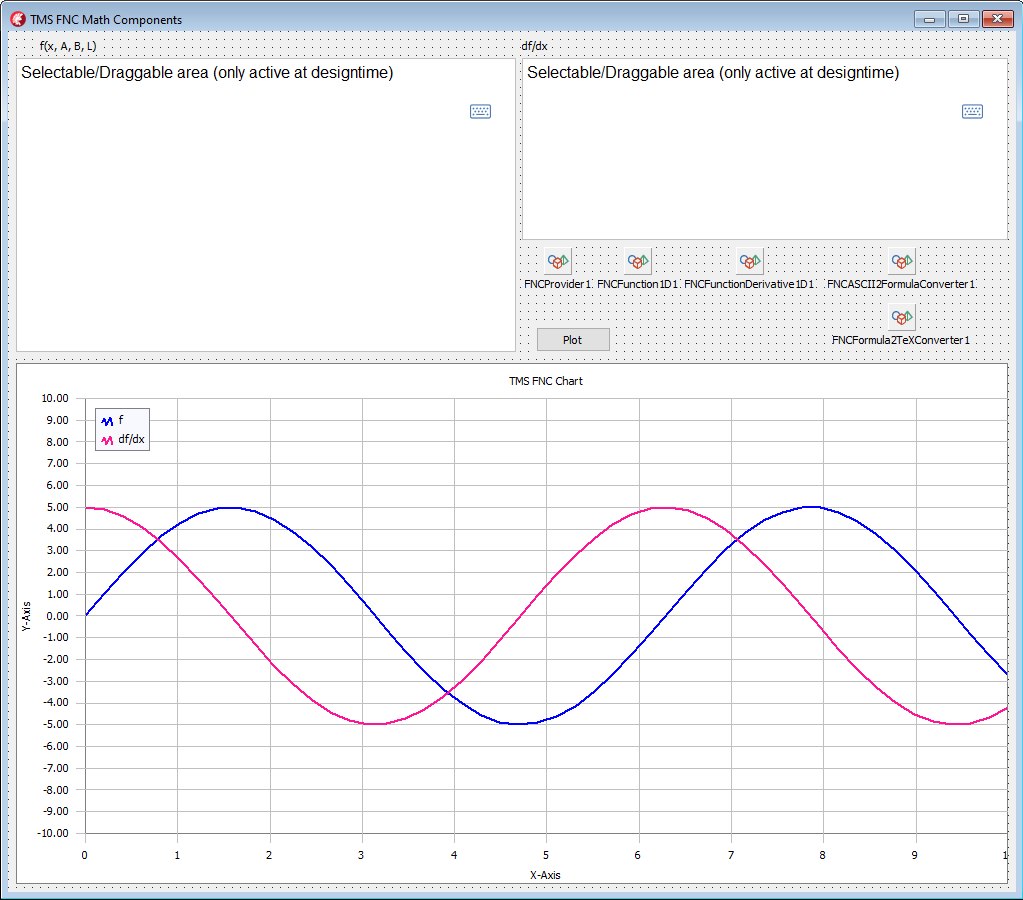Let’s start writing the code. First, we assigned the ‘MathFormat’ property of the first editor to the ‘mfASCIIMath’ value. This forces the component to present the output formula in ASCII Math format. Next, we created the following event handler for the ‘OnInput’ event of the component.
```procedure TForm1.TMSFNCWXMathEditor1Input(Sender: TObject; AResultString: string; AResultFormat: TTMSFNCWXMathFormat);
begin
FASCIIMathFormula:= AResultString;
end;```

When the formula in the editor is changed, we save its value in the ‘FASCIIMathFormula’ field of the form. After input, the user pushes the button to evaluate the derivative and the result is shown in the chart. The code of the ‘OnClick’ event handler is:

```procedure TForm1.Button1Click(Sender: TObject);
var
f, df, dftex, errmsg: string;
begin
f:= '';
dftex:= '';
errmsg:= '';

FNCASCII2FormulaConverter1.ASCIIFormula:= FASCIIMathFormula;

if FNCASCII2FormulaConverter1.Valid then
begin
f:= FNCASCII2FormulaConverter1.Formula;
FNCFunction1D1.Formula.Formula:= f;
if FNCFunction1D1.IsValid then
begin
df:= FNCFunctionDerivative1D1.Formula.Formula;
FNCFormula2TeXConverter1.Formula:= df;
if FNCFormula2TeXConverter1.Valid then
begin
dftex:= FNCFormula2TeXConverter1.TeXFormula;
end
else
errmsg:= FNCFormula2TeXConverter1.Error;
end
else
errmsg:= FNCFunction1D1.Formula.Error;
end
else
errmsg:= FNCASCII2FormulaConverter1.Error;

TMSFNCWXMathEditor2.Math:= dftex;

if not errmsg.IsEmpty then
ShowMessage(errmsg);
end;```
In the code above, the saved value of the ‘FASCIIMathFormula’ is assigned to the ‘ASCIIFormula’ property of the converter component. The component converts the formula in ASCII Math format to analytical expression and we assign this value to the ‘FNCFunction1D1’ component. The ‘FNCFunctionDerivative1D1’ component is connected with the function component, thus, it automatically evaluates the derivative on the function change. We convert the symbolic derivative expression to the TeX format with the ‘FNCFormula2TeXConverter1’ component and assign the formula to the second editor to show it.
Our application is ready. Now we can start it, input a formula, and get its derivative. Some examples of the formulae and their automatically evaluated derivatives are shown in the pictures below.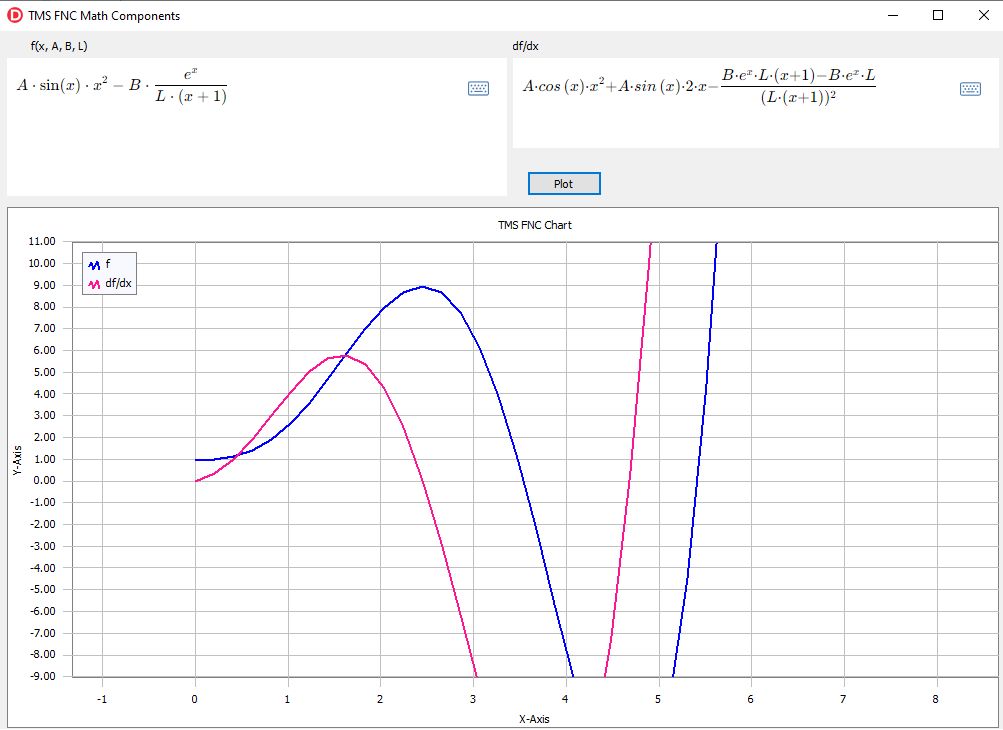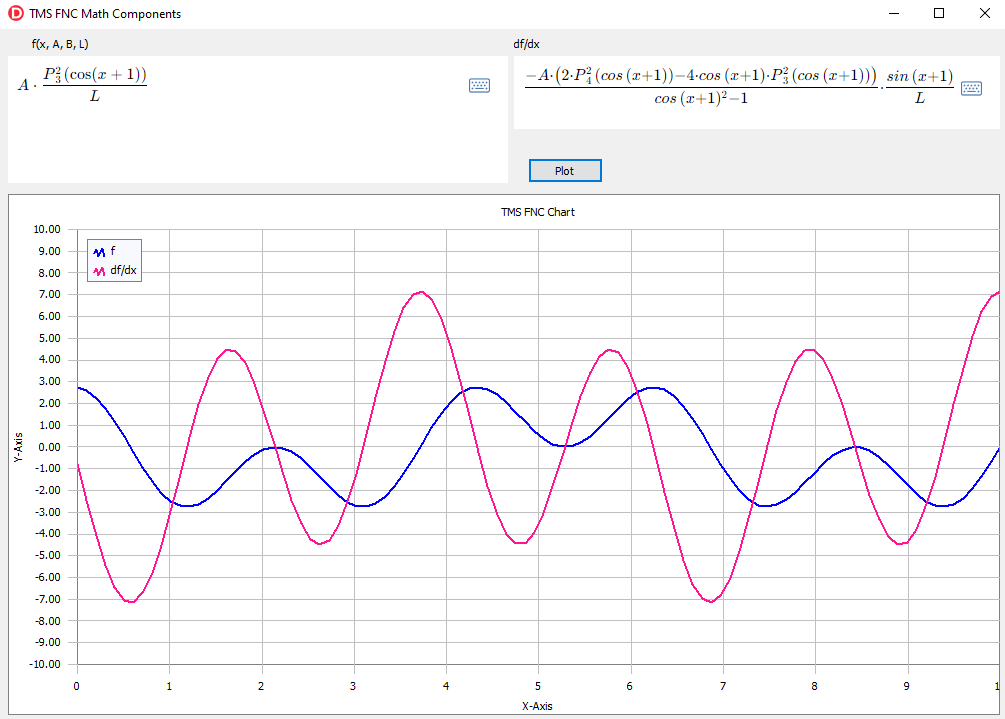You can download the full source code of the demo project from here. To use the application, you need the latest versions of TMS Analytics & Physics, TMS FNC Chart, and TMS FNC WX products.

Bruno Fierens

Bookmarks: